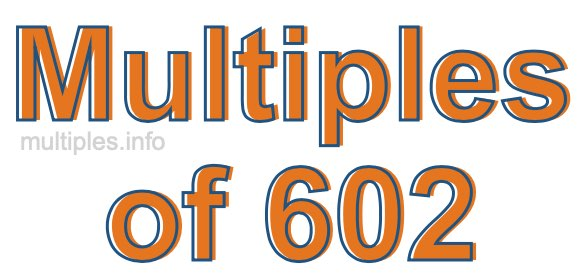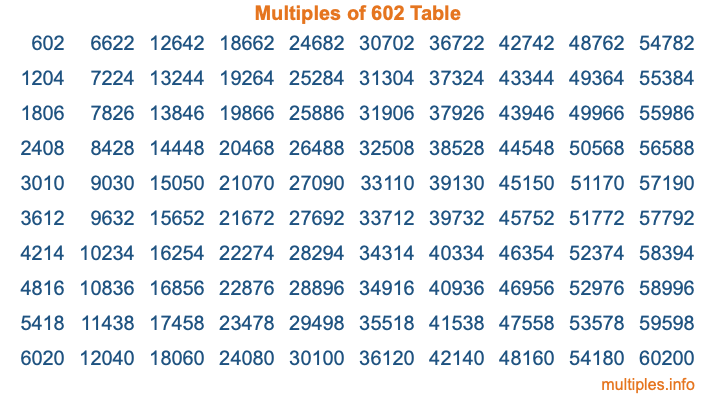Multiples of 602Welcome to the Multiples of 602 page. Here we will first teach you everything you will ever need to know about the multiples of 602, and then give you a study guide summary of everything we taught you to make sure you remember it all. Use this page to look up facts and learn information about the multiples of 602. This page will make you a multiples of six hundred two expert!

Definition of Multiples of 602
Multiples of 602 are all the numbers that when divided by 602 equal an integer. Each of the multiples of 602 are called a multiple. A multiple of 602 is created by multiplying 602 by an integer.

Therefore, to create a list of multiples of 602, you start with 1 multiplied by 602, then 2 multiplied by 602, then 3 multiplied by 602, and so on for as long as you want. Thus, the list of the first five multiples of 602 is 602, 1204, 1806, 2408, and 3010. To see a larger list of multiples of 602, see the printable image of Multiples of 602 further down on this page. We also have a category where you can choose any nth multiple of 602.

Multiples of 602 Checker
The Multiples of 602 Checker below checks to see if any number of your choice is a multiple of 602. In other words, it checks to see if there is any number (integer) that when multiplied by 602 will equal your number. To do that, we divide your number by 602. If the the quotient is an integer, then your number is a multiple of 602.

Is  a multiple of 602?

Least Common Multiple of 602 and ...
A Least Common Multiple (LCM) is the lowest multiple that two or more numbers have in common. This is also called the smallest common multiple or lowest common multiple and is useful to know when you are adding our subtracting fractions. Enter one or more numbers below (602 is already entered) to find the LCM.

Check out our LCM Calculator if you need more details about the Least Common Multiple or if you need the LCM for different numbers for adding and subtraction fractions.

nth Multiple of 602
As we stated above, 602 is the first multiple of 602, 1204 is the second multiple of 602, 1806 is the third multiple of 602, and so on. Enter a number below to find the nth multiple of 602.

th multiple of 602

Multiples of 602 vs Factors of 602
602 is a multiple of 602 and a factor of 602, but that is where the similarities end. All postive multiples of 602 are 602 or greater than 602. All positive factors of 602 are 602 or less than 602.

Below is the beginning list of multiples of 602 and the factors of 602 so you can compare:

Multiples of 602: 602, 1204, 1806, 2408, 3010, etc.

Factors of 602: 1, 2, 7, 14, 43, 86, 301, 602

As you can see, the multiples of 602 are all the numbers that you can divide by 602 to get a whole number. The factors of 602, on the other hand, are all the whole numbers that you can multiply by another whole number to get 602.

It's also interesting to note that if a number (x) is a factor of 602, then 602 will also be a multiple of that number (x).

Multiples of 602 vs Divisors of 602
The divisors of 602 are all the integers that 602 can be divided by evenly. Below is a list of the divisors of 602.

Divisors of 602: 1, 2, 7, 14, 43, 86, 301, 602

The interesting thing to note here is that if you take any multiple of 602 and divide it by a divisor of 602, you will see that the quotient is an integer.

Multiples of 602 Table
Below is an image of the first 100 multiples of 602 in a table. The table is in chronological order, column by column. The first column has the first ten multiples of 602, the second column has the next ten multiples of 602, and so on.The Multiples of 602 Table is also referred to as the 602 Times Table or Times Table of 602. You are welcome to print out our table for your studies.

Negative Multiples of 602
Although not often discussed or needed in math, it is worth mentioning that you can make a list of negative multiples of 602 by multiplying 602 by -1, then by -2, then by -3, and so on, to get the following list of negative multiples of 602:

-602, -1204, -1806, -2408, -3010, etc.

Multiples of 602 Summary
Below is a summary of important Multiples of 602 facts that we have discussed on this page. To retain the knowledge on this page, we recommend that you read through the summary and explain to yourself or a study partner why they hold true.

There are an infinite number of multiples of 602.

A multiple of 602 divided by 602 will equal a whole number.

602 divided by a factor of 602 equals a divisor of 602.

The nth multiple of 602 is n times 602.

The largest factor of 602 is equal to the first positive multiple of 602.

602 is a multiple of every factor of 602.

602 is a multiple of 602.

A multiple of 602 divided by a divisor of 602 equals an integer.

602 divided by a divisor of 602 equals a factor of 602.

Any integer times 602 will equal a multiple of 602.

Multiples of a Number
Here you can get the multiples of another number, all with the same attention to detail as we did for multiples of 602 on this page.

Multiples of
Multiples of 603
Did you find our page about multiples of six hundred two educational? Do you want more knowledge? Check out the multiples of the next number on our list!